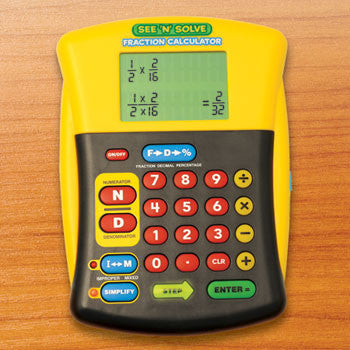See 'n' Solve Fraction Calculator | Freedom Day SalesSee 'n' Solve Fraction Calculator

Regular price \$39.99 Sale

Description:

• Let this unique calculator model solving fraction problems step-by-step
• Oversize LCD screen displays each step
• shows amounts in mixed number and improper fraction form, and answers in reduced form
• Easily input addition, subtraction, multiplication and division equations or convert between fractions, decimals and percents
• Features normal calculator mode
• Includes 12-page Activity Guide and quick reference stickers
• Measures approximately 4 1/2"L x 6 1/4"H.
• Requires 2 AA batteries, not included.